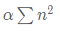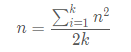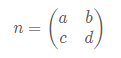# Formulas and equations

For some assignments you might want to add mathematical expressions to your texts. This is very easy in Quarterfall. You simply write a description of the formula between $symbols. So for example you can write$\alpha \sum n^2$which results inYou can either add the formula inline by putting it between single$ signs, as in the example above, or centered on a separate line by putting the formula on a separate line between $$signs, like this:$$
n = \frac{\sum_{i=1}^k n^2}{2k}
$$which results inNot only is the formula centered but it is also shown in a less condensed way. Here is another example:$$
n =\begin{pmatrix} a & b \\ c & d \end{pmatrix}


which results inWe use KaTeX to render the formulas. This supports most of the TeX/LaTeX formulas, which are also used in other packages like MatLab. This includes many special symbols and formula building commands. Here are the basics:

• Use ^ for a superscript and _ for a subscript. If you need more than one symbol as a subscript, put it between { and }. Also symbols like \sum, \int, and \forall can have superscripts and subscripts.
• For greek symbols, use a backslash followed bu the name, like \alpha or \Alpha to get the uppercase version of it. This also works for many other symbols like \log or \sin.
• Some functions change the way things look. The argument is between { and }. For example \bf{abc} make it bold and \it{abc} makes it italic. \overline{abc} puts a line over the argument, \sqrt{abc} show a square root symbol, et cetera.
• Some functions have two arguments, like \frac{a}{b} that was used above.
• If you want to put normal text in a formula, use \text{this is normal text}.

For a complete set of supported functions see the KaTeX overview

Note that you can immediately see a preview of what your formulas look like while editing the assignment.